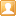# [功能实施] 二次开发-数据模型-工单数据模型-简单变更管理匿名  发表于 2020-12-18 10:07:04 |阅读模式

• 补丁的安装
• 系统配置的更改
• 操作系统更新
• 软件安装

• 变更实现者计划并实施更改
• 变更管理员跟进更改
• 变更经理批准更改

 名称 类型 强制性的吗？ 编号 字母数字字符串 是 组织 一个（n）组织的外键 是 状况 可能的值：已批准，已分配，已关闭，新建，计划中，已拒绝 没有 标题 字母数字字符串 是 描述 多行字符串 是 提交人 一个人的外键 是 球队 团队的外键 没有 处理人员 一个人的外键 没有 变更经理 一个人的外键 没有 类别 可能的值：应用，硬件，网络，其他软件，系统 没有 拒绝原因 多行字符串 没有 回退计划 多行字符串 没有 父变更 一个（n）变更的外键 没有 创建日期 日期和时间（年月日hh：mm：ss） 没有 开始日期 日期和时间（年月日hh：mm：ss） 没有 结束日期 日期和时间（年月日hh：mm：ss） 没有 最后更新 日期和时间（年月日hh：mm：ss） 没有 批准日期 日期和时间（年月日hh：mm：ss） 没有 截止日期 日期和时间（年月日hh：mm：ss） 没有 断电 可能的值：是，否 没有

 标签 描述 配置项 此工单受影响的所有配置项目 联络人 与此工单链接的所有联系人 工作订单 工单的所有工作订单 相关要求 与此变更关联的所有用户请求 相关问题 与此变更相关的所有问题 换小孩 与此变更链接的所有子更改新 已分配 计划 拒绝 已批准 已关闭 编号 R/O R/O R/O R/O R/O R/O 组织 M M M R/O R/O R/O 状况 R/O R/O R/O R/O R/O R/O 标题 M M M R/O R/O R/O 描述 M M M R/O R/O R/O 提交人 M M R/O R/O R/O 球队 H M M R/O R/O R/O 处理人员 H M M R/O R/O R/O 变更经理 H M M R/O R/O R/O 类别 R/O R/O 拒绝原因 H H H M R/O R/O 回退计划 M R/O R/O 父变更 R/O R/O 创建日期 R/O R/O R/O R/O H R/O 开始日期 M R/O R/O R/O 结束日期 M R/O R/O R/O 最后更新 R/O R/O R/O R/O R/O R/O 批准日期 H H H R/O R/O R/O 截止日期 H H H H H R/O 断电 H H M R/O R/O R/O

• H：隐藏
• RRO：只读
• M：必填

 本版积分规则 回帖并转播 回帖后跳转到最后一页
ITIL(R) is a registered trademark of AXELOS Limited, used under permission of AXELOS Limited. The Swirl logo is a trademark of AXELOS Limited, used under permission of AXELOS Limited. All rights reserved.|ITIL ( 粤ICP备11099876号 )|appname

GMT+8, 2023-9-26 21:27 , Processed in 0.127066 second(s), 25 queries .